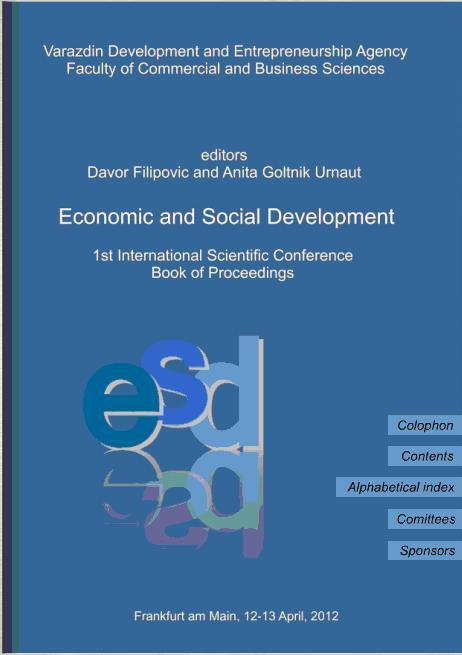• A
• A
• A
• ABC
• ABC
• ABC
• А
• А
• А
• А
• А
Regular version of the site

## The method of determining importance of criteria in a multicriteria decision problem

P. 441-452.
Perminov G. I.

This abstract offers a method for ranking alternatives in a decison making problem. It determines importance of the criteria with help of factor analysis. Though the alternatives are evaluated by each of the criteria by a group of experts, the weights for the criteria are to be found with the help of factor analysis.

The algorithm of the method is as follows:

1. Under the constraint that the problem handles several evaluation criteria, several items to compare (alternatives) and several experts to give their evaluation.

2. Find the principal components that replace the input criteria implicitly.

3. To find the final mark for each of the alternatives the marks given by experts are multiplied with the regression coefficients, found in the step 2.

4. The final marks are represented in axes „crieria“ and „mark“ so that each alternative is described with a curve (trajectory). These curves represent the map of graded alternatives. Depending on the problem to be solved (min or max,) a record for each main criteria is to be found.

5. With help of special deviation measure procedures (Minkowski, Chebyshev e.t.s) a  matrix of deviations from ideal solution is to be built.

6. The alternatives are to be rated in accordance to the deviation from the ideal trajectory.

To prove the effectiveness of the method it was applied to a problem for 5 alternatives, 3 experts and 38 evaluation criteria. The problem was also solved with the help of most popular method of Weighted Sum Model (WSM) and TOPSIS method. The problem was also being solved by finding the geometric mean for each alternative. The results for approaches were compared and the method, offered in this abstrat, proved itself as a feasible one.

### In bookEdited by: D. Fillpovic, A. Goltnik. Varazdin: Varazdin development and Entepreneurship Agency, 2012.